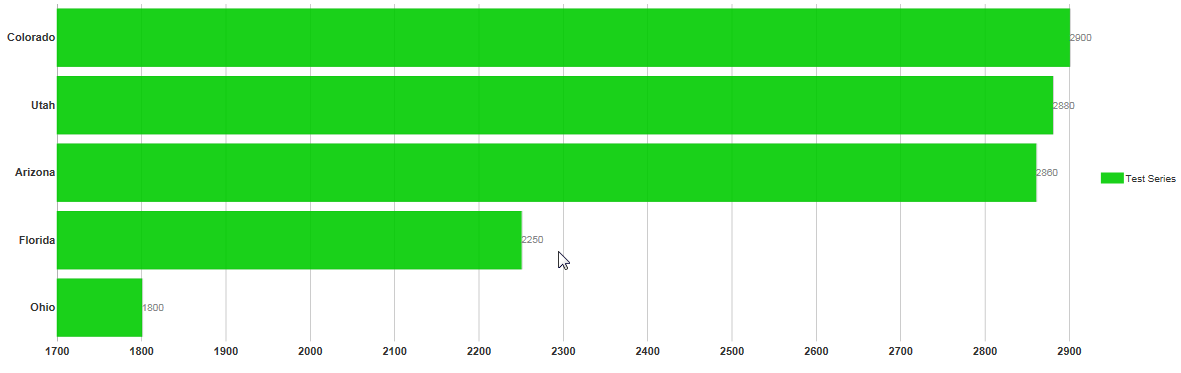WijBarChart displays the chart data in the form of static bars, that displays the YAxis data. Here in this blog, we will discuss how to animate bars of Wijmo BarChart on hovering mouse.

### Implementation

The basic idea is to change the position of the bar, when the mouse is hovered over or out of the bar. To do this, we will use the hover event of the bartracker class. Here is the complete code :

``````  //Code to callout bars
var oldx = 0;
var oldindex = -1;
\$(\".bartracker\").hover(function (e) {
var target = \$(e.target);
if (target.data('owner')) {
target = target.data('owner');
}
var data = target.data('wijchartDataObj');
if (data) {
var sbar = data.bar;
oldx = sbar.attrs.x;
oldindex = data.index;
var newx = sbar.attrs.x + 20;
sbar.attr(\"x\", newx);
}
}, function (e) {
if (oldx != 0 && oldindex != -1) {
var obar = \$(\"#wijbarchart\").wijbarchart(\"getBar\", oldindex);
obar.attr(\"x\", oldx);
}
});
``````

### Conclusion

Here is how our BarChart would look like after the above implementation :